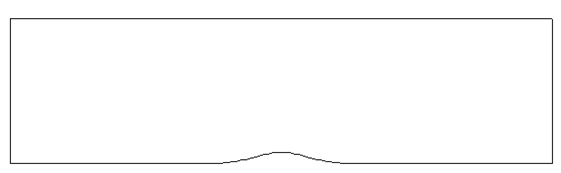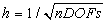Problem C1.1. Inviscid Flow through a Channel with a Smooth Bump

Overview

This problem is aimed at testing high-order methods for the computation of internal flow with a high-order curved boundary representation. In this subsonic flow problem, the geometry is smooth, and so is the flow. Entropy should be a constant in the flow field. The L2 norm of the entropy error is then used as the indicator of solution accuracy since the analytical solution is unknown.

Governing Equations

The governing equation is the 2D Euler equations with a constant ratio of specific heats of 1.4.

Flow Conditions

The inflow Mach number is 0.5 with 0 angle of attack.

Geometry

The computational domain is bounded between x = -1.5 and x = 1.5, and between the bump and y = 0.8, as shown in Figure 1.1. The bump is defined asFigure 1.1 Channel with a Smooth Bump

Boundary Conditions

Left boundary: subsonic inflow

Right boundary: subsonic exit

Top boundary: symmetry

Bottom boundary: slip wall

Requirements

1.     Start the simulation from a uniform free stream with M = 0.5 everywhere, and monitor the L2 norm of the density residual. Compute the work units required to achieve a steady state. Use the following non-dimensional entropy error as the accuracy indicator2.     Perform this exercise for at least three different meshes and with different orders of accuracy to assess the performance of high-order schemes of various accuracy.

3.     Plot the L2 entropy error vs. work units to evaluate efficiency, and L2 entropy error vs. length scale to assess the numerical order of accuracy.

4.     Submit two sets of data to the workshop contact for this case

a.      Error vs work units for different h and p

b.     Error vsfor different h and p